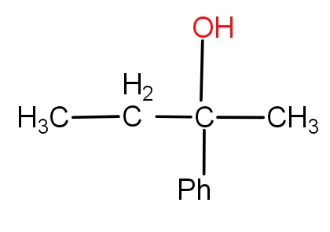#Can not be prepared by :a.) HCHO + $PhCH(C{H_3})C{H_2}MgX$b.) $PhCOC{H_2}C{H_3}$+$C{H_3}MgX$c.) $PhCOC{H_3}$+$C{H_3}C{H_2}MgX$d.) $C{H_2}C{H_3}COC{H_3}$+PhMgXVerified
179.1k+ views
Hint: The Grignard reagent reacts with formaldehyde to form primary alcohol while with all other aldehydes, it forms second alcohol. And further, the Grignard reagent with ketones yields tertiary alcohol. On the basis of this, we see that the options that have a group other than ketone will be the correct answer.

Complete step by step answer :
We know that RMgX is Grignard reagent. The Grignard reagent reacts with formaldehyde to form primary alcohol while with all other aldehydes, it forms second alcohol. And further, the Grignard reagent with ketones yields tertiary alcohol. The product given to use is tertiary alcohol. So, the answer would be the reaction of Grignard reagent with ketone. Now, let us see the options given to us.
-So, the first option is HCHO and $PhCH(C{H_3})C{H_2}MgX$.
-The formaldehyde is an aldehyde. It reacts with $PhCH(C{H_3})C{H_2}MgX$ which is a Grignard reagent to give primary alcohol. So, it will not give the desired product. Thus, it would be the correct answer.
-The second option we have is $PhCOC{H_2}C{H_3}$ and $C{H_3}MgX$. Here, the $C{H_3}MgX$ is a Grignard reagent and the other substrate is the ketone. So, we will get tertiary alcohol. This will give the desired product. So, this can-not be the answer.
-The third option is $PhCOC{H_3}$ and $C{H_3}C{H_2}MgX$. Here, also; ketone is present. So, it will yield the tertiary alcohol and will form the desired product. So, this can-not be the answer.
-The fourth option given is $C{H_2}C{H_3}COC{H_3}$ and PhMgX. Even here, ketone is present. So, it will yield the tertiary alcohol and will form the desired product. So, this can-not be the answer.

So, the correct answer is option a.).

Note: It must be noted that Grignard reagent is an organometallic compound. It has a direct metal carbon bond. It is used to form carbon-carbon bonds. Water and air can destroy the Grignard reagent. So, the reaction with the Grignard reagent is done in an inert atmosphere.